Most versatile effective function and unique to Google Spreadsheets. I am documenting this function to understand it properly and for those who do not have programming background. I do not have previous experience with query. i will try to illustrate with examples.

Google Spreadsheet query is designed to be similar to SQL  with few exceptions. it is a subset of  SQL with a few feature of its own. if you are familiar with SQL it will be easy to learn.

The Syntax of the function is as follow:

DATA: it can be columns(A:C open ranges) of data you want to query, range of cells such as A1:C10, result of function such as importrange, index etc.,

QUERY: It is similar to SQL with small exceptions there is no FROM clause in the this since DATA itself is acting like a FROM clause.

Notes:

1. column headers have to capital letter such A, B, C if you are picking up the raw data with in the same spreadsheet.

2. if you are using the array formulas to manipulate the data (Index, Filter, importrange to name a few) then column headers will Col1, Col2, Col3 etc. observe i have used C capital letter in Col1. this is syntax you have to follow this otherwise you will get a parse error

3. parse error:  an error of language resulting from code that does not conform to the syntax of the programming language; “syntax errors can be recognized at compilation time”

4. Data types: supports data types are string, number, boolean, date, datetime and timeof day. all values of the column will have a data type that matches the column type or a null value

5. The syntax of the query language is composed of the following clauses. Each clause starts with one or two keywords. All clauses are optional. Clauses are separated by spaces. The order of the clauses must be as follows:

 Clause Useage select Select which columns to return, and in what order, if omitted, all the table’s columns are returned, in their default order where Return only rows that match a condition. if omitted, all rows are returned. group by Aggregates values across rows pivot Transforms distinct values in columns into new column order by sorts rows by values in columns limit Limits the number of returned rows offset skips a given number of first rows label sets column labels format formats the values in certain columns using given formatting patterns options sets additional options

now let try to understand these clauses with an example

6. our data set like this

s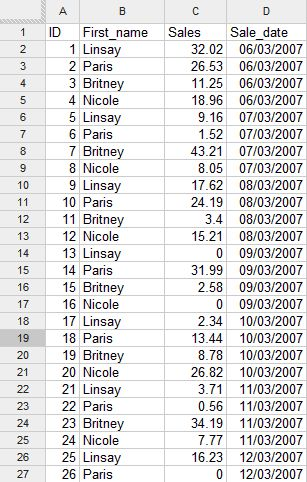7. try this formula

=QUERY(A:D;”select A,B”;1) the result will be as show in the below image

8.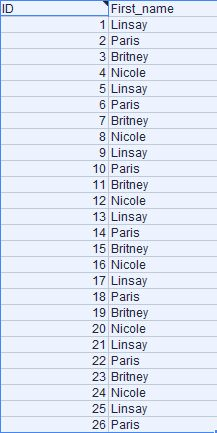We have some special keywords called functions, Functions are bits of code that perform an operation on a value or values. The first we will see is to perform a mathematical operation on a column. We will see Sum function which by totaling the values in a column designated by parentheses.

suppose we want to Sum Column C where the Column B is Nicole

=QUERY(A:D;”select B, Sum(C) where B = ‘Nicole’ group by B”;1)

9. the result will be

10.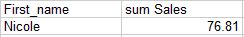Note:

(1) you have to use S capital in the Sum followed by column you want sum in parentheses

(2) whenever you are using the group by clause same column has to be selected in the select clause otherwise you may get value error

(3) condition you want the check in the column B has to be in single quote

this can be sorted using the order by clause

=QUERY(A:D;”select B, Sum(C) where B <>” group by B order by B asc”;1)

the result will be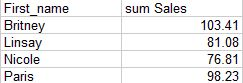Note:

(1) observe the column 1 has been sorted in ascending order

(2) to get the the descending order you can use the desc instead of asc

11. you can also sort the based on the result of the Sum(C)

=QUERY(A:D;”select B, Sum(C) where B <>” group by B order by Sum(C) desc”;1)

the results will be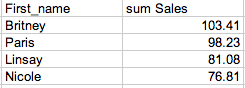Note:

(1) we have sorted data by descending order based on Sum of sales

(2) you have to follow the order of clause listed above

We can also limit the results top 3 or top 2 using the limit clause

=QUERY(A:D;”select B, Sum(C) where B <>” group by B order by Sum(C) desc limit 3″;1)We can also the name the column sum Sales as Top 3 sales

=QUERY(A:D;”select B, Sum(C) where B <>” group by B order by Sum(C) desc limit 3 label Sum(C) ‘Top 3 Sales'”;1)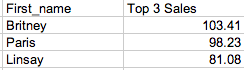We can also pivot the data based on date in the D column.

=QUERY(A:D;”select B, Sum(C) where B <>” group by B pivot D”;1)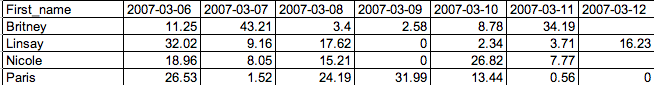Note:

(1) You might have observed the column D is not selected the select clause

(2) Date are formatted in yyyy-mm-dd format

(3) pivot is unique to google Sheets Query function

12. In the next blog post we will see how to manipulate data using the cell value